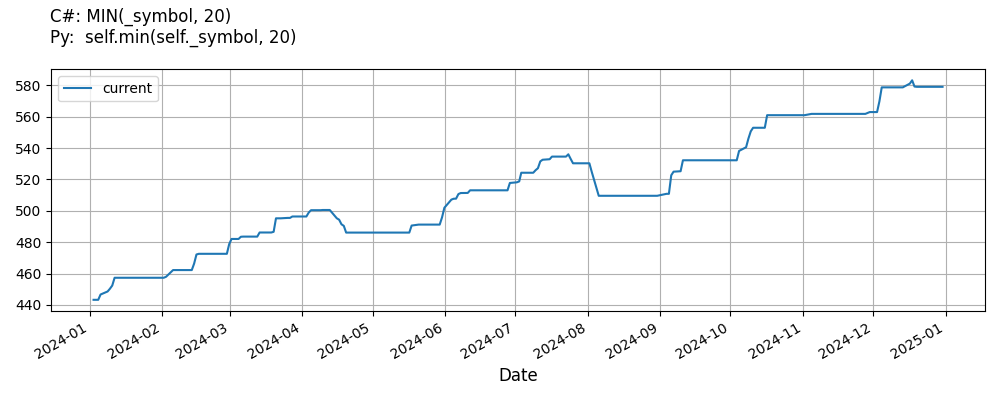# Supported Indicators

## Minimum

### Introduction

This indicator represents an indicator capable of tracking the minimum value and how many periods ago it occurred

To view the implementation of this indicator, see the LEAN GitHub repository.

### Using MIN Indicator

To create an automatic indicators for Minimum, call the MIN helper method from the QCAlgorithm class. The MIN method creates a Minimum object, hooks it up for automatic updates, and returns it so you can used it in your algorithm. In most cases, you should call the helper method in the Initialize method.

public class MinimumAlgorithm : QCAlgorithm
{
private Symbol _symbol;
private Minimum _min;

public override void Initialize()
{
_min = MIN(_symbol, 20);
}

public override void OnData(Slice data)
{
{
// The current value of _min is represented by itself (_min)
// or _min.Current.Value
Plot("Minimum", "min", _min);
// Plot all properties of min
Plot("Minimum", "periodssinceminimum", _min.PeriodsSinceMinimum);
}
}
}
class MinimumAlgorithm(QCAlgorithm):
def Initialize(self) -> None:
self.min = self.MIN(self.symbol, 20)

def OnData(self, slice: Slice) -> None:
# The current value of self.min is represented by self.min.Current.Value
self.Plot("Minimum", "min", self.min.Current.Value)
# Plot all attributes of self.min
self.Plot("Minimum", "periodssinceminimum", self.min.PeriodsSinceMinimum)


The following reference table describes the MIN method:

### MIN()1/1

            Minimum QuantConnect.Algorithm.QCAlgorithm.MIN (
Symbol                           symbol,
Int32                            period,
*Nullable<Resolution>      resolution,
*Func<IBaseData, Decimal>  selector
)


Creates a new Minimum indicator to compute the minimum value.

If you don't provide a resolution, it defaults to the security resolution. If you provide a resolution, it must be greater than or equal to the resolution of the security. For instance, if you subscribe to hourly data for a security, you should update its indicator with data that spans 1 hour or longer.

You can manually create a Minimum indicator, so it doesn't automatically update. Manual indicators let you update their values with any data you choose.

Updating your indicator manually enables you to control when the indicator is updated and what data you use to update it. To manually update the indicator, call the Update method with time/number pair, or an IndicatorDataPoint. The indicator will only be ready after you prime it with enough data.

public class MinimumAlgorithm : QCAlgorithm
{
private Symbol _symbol;
private Minimum _min;

public override void Initialize()
{
_min = new Minimum(20);
}

public override void OnData(Slice data)
{
if (data.Bars.TryGeValue(_symbol, out var bar))
{
_min.Update(bar.EndTime, bar.Close);
}

{
// The current value of _min is represented by itself (_min)
// or _min.Current.Value
Plot("Minimum", "min", _min);
// Plot all properties of min
Plot("Minimum", "periodssinceminimum", _min.PeriodsSinceMinimum);
}
}
}
class MinimumAlgorithm(QCAlgorithm):
def Initialize(self) -> None:
self.min = Minimum(20)

def OnData(self, slice: Slice) -> None:
bar = slice.Bars.get(self.symbol)
if bar:
self.min.Update(bar.EndTime, bar.Close)

# The current value of self.min is represented by self.min.Current.Value
self.Plot("Minimum", "min", self.min.Current.Value)
# Plot all attributes of self.min
self.Plot("Minimum", "periodssinceminimum", self.min.PeriodsSinceMinimum)


To register a manual indicator for automatic updates with the security data, call the RegisterIndicator method.

public class MinimumAlgorithm : QCAlgorithm
{
private Symbol _symbol;
private Minimum _min;

public override void Initialize()
{
_min = new Minimum(20);
RegisterIndicator(_symbol, _min, Resolution.Daily);
}

public override void OnData(Slice data)
{
{
// The current value of _min is represented by itself (_min)
// or _min.Current.Value
Plot("Minimum", "min", _min);

}
}
}
class MinimumAlgorithm(QCAlgorithm):
def Initialize(self) -> None:
self.min = Minimum(20)
self.RegisterIndicator(self.symbol, self.min, Resolution.Daily)

def OnData(self, slice: Slice) -> None:
# The current value of self.min is represented by self.min.Current.Value
self.Plot("Minimum", "min", self.min.Current.Value)



The following reference table describes the Minimum constructor:

### Minimum()1/2

            Minimum QuantConnect.Indicators.Minimum (
int  period
)


Creates a new Minimum indicator with the specified period.

### Minimum()2/2

            Minimum QuantConnect.Indicators.Minimum (
string  name,
int     period
)


Creates a new Minimum indicator with the specified period.

### Visualization

The following image shows plot values of selected properties of Minimum using the plotly library.You can also see our Videos. You can also get in touch with us via Discord.